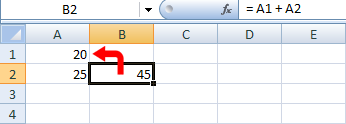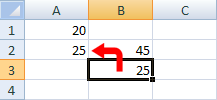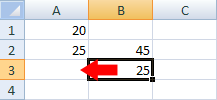Home and Learn: Microsoft Excel Course

# Excel \$ cell reference

An important difference in Excel spreadsheets is between absolute cell references and relative cell references. To see what this is all about, we'll create a simple spreadsheet. This will illustrate relative cell references, which is what we've been using so far. In other words, we haven't been using the dollar (\$) sign yet in our formulas. We'll remedy this now.

So open up Excel and enter the same values as in the image below:In cell B2, you need the following formula:

= A1 + A2

What do you think would happen if we copied an pasted the formula from B2 to cell B3? Let's see:

• Click inside cell B2 to highlight it
• Click on cell B2 with your right mouse button, and select Copy from the menu that appears
• Now click into cell B3
• Again, right click the cell to get the menu. But this time click Paste
•Cell now says 25! We were trying to work out what 20 + 25 was, and have the wrong answer. So why did Excel put 25 into cell B3 and not 45?

With cell B3 still highlighted, look at the formula bar at the top of Excel. You should see this formula:

= A2 + A3

Click into B2, however, and the formula is this:

= A1 + A2

The problem is due to cell referencing. When you clicked Copy from the menu, Excel didn't only copy the formula. It took at look at where the cells were in the formula, relative to the B2 cell, and copied this as well. From B2, the first cell reference (A1) is up one row, and left 1 column (the red arrow below):The second cell reference (A2) is one column to the left of cell B2:When you clicked into cell B3 and selected Paste from the menu, Excel was not only pasting the formula, it was pasting this "up 1, left 1". Take a look at the two images below. We're now starting at cell B3. Have a look at where the two red arrows are pointing now.

The first cell reference:The second cell reference:So the first red arrow is pointing to cell A2, and the second red arrow is point to cell A3. This is what was copied. Excel then took the formula to mean this:

= A2 + A3

But it should have been this:

= A1 + A2

If you want the correct answer in cell B3, you have to stop Excel from using this Relative Cell Referencing that it's currently doing. What you need is Absolute Cell Referencing.

Absolute cell referencing involves nothing more than placing a dollar symbol ( \$ ) before each letter and number.

Click inside of cell B2 on your spreadsheet, and change the formula to this:

= \$A\$1 + \$A\$2

Now copy and paste it over to cell B3 again. You should have the correct answer, this time:Excel will use Absolute Formula in its own calculation, so it's worth getting used to them. But to recap:

• If you need to copy and paste formulas, use Absolute cell references
• Absolute referencing means typing a dollar symbol before the numbers and letters of each cell reference (You can mix absolute and relative cell references, though).
• In the next part, we'll take a look at Named Ranges in Excel.

<--Back to the Excel Contents Page

Email us: enquiry at homeandlearn.co.uk